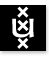Advanced Topics in Set Theory 2005/2006; 1st Semester Institute for Logic, Language & Computation Universiteit van Amsterdam
Instructor: Dr Benedikt Löwe
Vakcode: MolATST6
Time: Wednesday, 17-19
Place: P.016
Course language: English
Teaching Assistant: Brian Semmes
Intended Audience: M.Sc. students of Logic and Mathematics
Prerequisites: This course assumes knowledge comparable to the course "Axiomatic Set Theory".
Literature: Kenneth Kunen, Set Theory, An Introduction to Independence Proofs, North-Holland 1980 [Studies in Logic and the Foundations of Mathematics 102]
amazon.de: € 83.50
Elsevier: € 76.

In this class, we shall cover the important technique of forcing. Our motivation is Hilbert's First Problem: the question whether there is a bijection between the first uncoutable ordinal omega1 and the set of real numbers (this statement is called the continuum hypothesis CH). In the early 1960s, Paul Cohen developed the technique of forcing that allows to construct models of set theory in which CH is true and those in which it is false.

This class will be much more metamathematical than other set theory classes and will require some preparatory work on models of set theory before we can start developing the theory of forcing.

Grading is based on homework assignments.

Classes:

• September 7, 2005. Class cancelled because of the Annual ILLC Boat Trip. All students enrolled in the class are cordially invited to join us for the boat trip.
• September 14, 2005. (First Lecture.) General introduction. The continuum problem. A very informal introduction to the methodology of forcing. Relativization of formulas.
Homework: Exercises IV.(6) and IV.(8).
• September 21, 2005. (Second Lecture.) Literature on higher set theory. Absoluteness.
Homework: PDF file.
• September 28, 2005. (Third Lecture.) More on absoluteness. Absoluteness of recursive definitions. The Hkappa and inaccessible cardinals. The consistency strength hierarchy.
Homework: Exercises IV.(10) and IV.(30).
• October 5, 2005. (Fourth Lecture.) Lévy Reflection Theorems. Mostowski Collapse (without proof). Defining Definability (without any details). Definition of OD and HOD.
Homework: Exercises III.(10), III.(14) and V.(5) (pages 108 and 163 in Kunen).
• October 12, 2005. (Fifth Lecture.) The constructible hierarchy. The Axiom of Constructibility V=L. The Condensation Lemma. Notation for partial orders in forcing: stronger than, chain conditions, etc.. Examples. Definition of MA(kappa).
Homework: Exercises VI.(2), VI.(3), II.(8), and II.(16) (pages 180, 87 and 88 in Kunen).
• October 19, 2005. (Sixth Lecture.) More examples of partial orders. MA(aleph0). P-names. The generic extension. Minimality of the generic extension.
Homework: Exercises VII.A1 (p.237), VII.A5, VII.A6, VII.A7, VII.A10 (p.238), VII.B1 (p.239). Due on November 2, 2005!
• October 26, 2005. No Class (Exam week)
• November 2, 2005. (Seventh Lecture.) Proof of some ZFC axioms in M[G]. The (semantic) forcing relation. The syntactic forcing relation. The Forcing Lemma.
Homework: Exercises VII.A12 (p.239), VII.B5 (p.240).
• November 9, 2005. (Eighth Lecture.) The Forcing Lemma (ctd.). ZFC in M[G].
Homework: PDF file.
• November 16, 2005. (Ninth Lecture.) Consistency proofs with forcing. Collapsing cardinals. Adding reals. Cardinal preservation. Every c.c.c. forcing preserves cardinals. Delta System lemma. Adding kappa many Cohen reals is c.c.c.
Homework: Exercises II.1 (p.86), VII.A2 (p.237).
• November 23, 2005. (Ninth Lecture.) Preserving cofinalities and preserving regularity. Computing the exact cardinality of the continuum in the Cohen model. Adding subsets of larger cardinals.
Homework: Read p.234-236 of Kanamori's book on Prikry forcing. Find out why Prikry forcing is an example of a forcing that preserves cardinals but not cofinality (Exercise 18.5). Also do Kunen's exercise VII.(G1) on p.246.
• November 30, 2005. (Tenth Lecture.) Simultaneously changing 2aleph-0 and 2aleph-1. lambda-closed forcing and preservation of cardinals. Collapses. Preservation of aleph1 and reals. Complete embeddings.
Homework: Exercises VII.C1, VII.C2, VII.C3, VII.C4 (p.242), VII.G2, VII.G5 (p.246). Due on December 14, 2005!
• December 7, 2005. Class cancelled
• December 14, 2005.
• December 21, 2005. No Class (Exam week)

Last update : November 30th, 2005